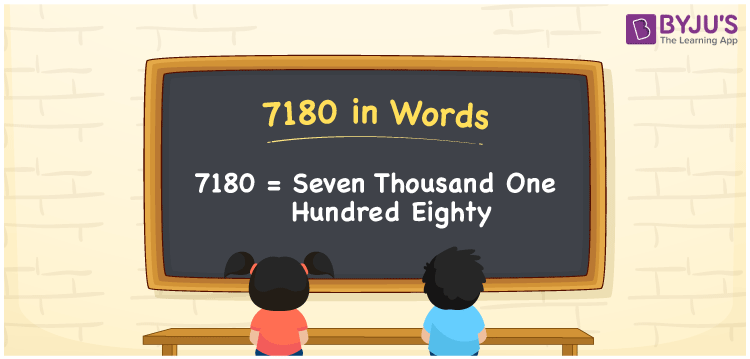# 7180 in Words

7180 in words can be written as Seven Thousand One Hundred Eighty. Students will be able to learn the conversion of 7180 in words which will help them understand the applications of numbers in our daily lives. If you buy a watch for Rs. 7180, then you can say that “I have bought a watch for Seven Thousand One Hundred Eighty Rupees”. The  number 7180 can be written in words using the English alphabet. The numbers in words can be grasped easily by the students using the resources given at BYJU’S. 7180 in English can be read as “Seven Thousand One Hundred Eighty”.

 7180 in words Seven Thousand One Hundred Eighty Seven Thousand One Hundred Eighty in Numbers 7180

## 7180 in English Words## How to Write 7180 in Words?

Students will learn about the conversion of 7180 into words from place value charts. The number 7180 has four digits. For 7180, the place value chart is prepared in a table form to help students understand it effectively.

 Thousands Hundreds Tens Ones 7 1 8 0

7180 in expanded form is explained in brief here:

7 × Thousand + 1 × Hundred + 8 × Ten + 0 × One

= 7 × 1000  + 1 × 100 + 8 × 10 + 0 × 1

= 7000 + 100 + 80

= 7180

= Seven Thousand One Hundred Eighty

Therefore, 7180 in words is written as Seven Thousand One Hundred Eighty.

7180 is a natural number that precedes 7181 and succeeds 7179.

7180 in words – Seven Thousand One Hundred Eighty

Is 7180 an odd number? – No

Is 7180 an even number? – Yes

Is 7180 a perfect square number? – No

Is 7180 a perfect cube number? – No

Is 7180 a prime number? – No

Is 7180 a composite number? – Yes

## Frequently Asked Questions on 7180 in Words

Q1

### How do you write 7180 in words?

7180 can be written as “Seven Thousand One Hundred Eighty” in words.
Q2

### Is 7180 an even number?

7180 is an even number because it is divisible by 2.
Q3

### How can Seven Thousand One Hundred Eighty be written in numbers?

Seven Thousand One Hundred Eighty can be written in numbers as 7180.